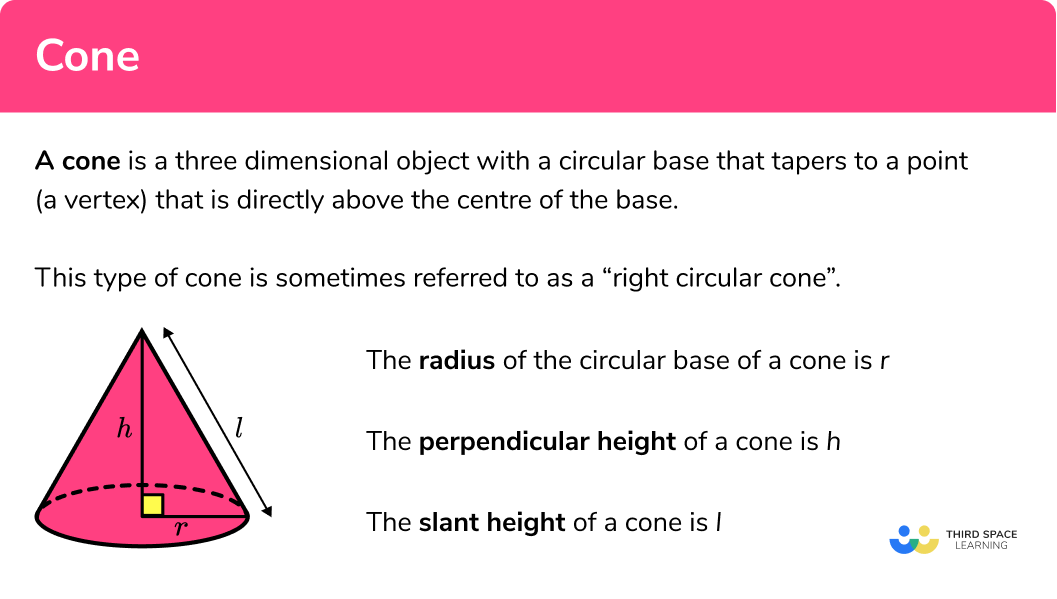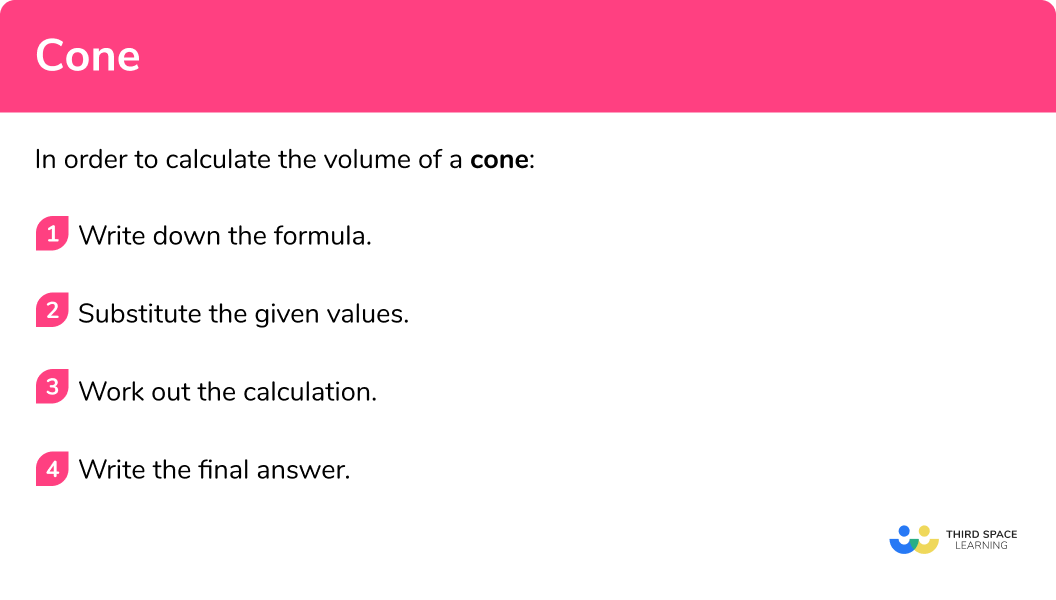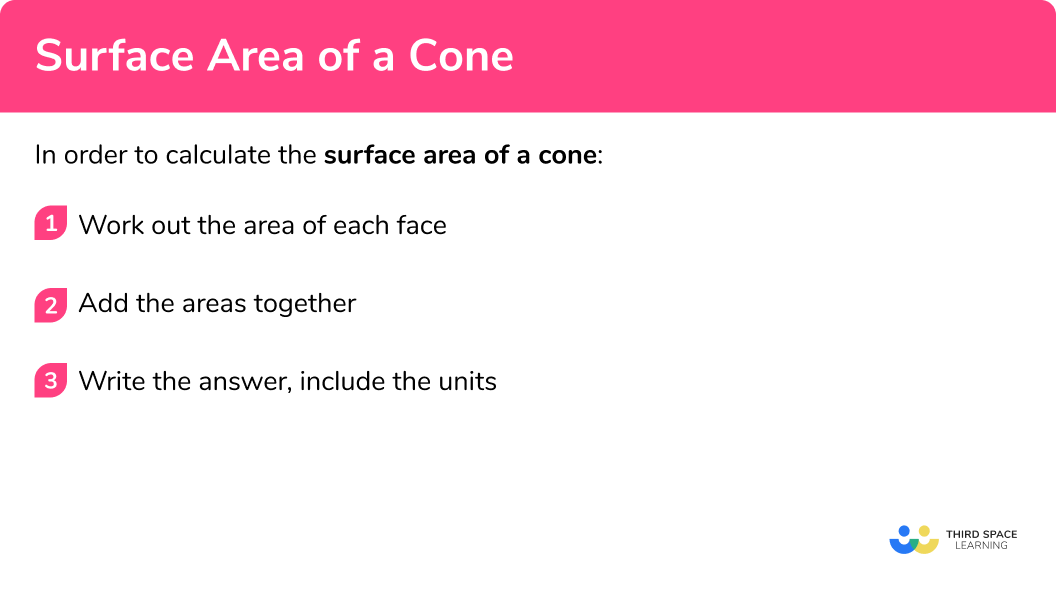# Cone

Here we will learn about cones, including how to find the volume of a cone and how to find the surface area of a cone.

There are also cone worksheets based on Edexcel, AQA and OCR exam questions, along with further guidance on where to go next if you’re still stuck.

## What is a cone?

A cone is a three dimensional object with a circular base that tapers to a point that is directly above the centre of the base. This point is called the vertex or apex of the cone.

Cone shapes that you are used to in real-life would be ice cream cones or traffic cones. This type of cone is sometimes referred to as a “right circular cone” or “right cone”. There are also oblique cones where the apex is not directly above the centre of the base, and also cones that have an ellipse as a base rather than a circle.

Here are the important properties of a cone that you will need for GCSE Mathematics.

The radius of the base of the cone is r

The perpendicular height of the cone is h , this is the height at a right angle to the base.

The slant height of the cone is l

Cones feature in A level Further Maths in a topic called Conic Sections. This topic explores the links between cones and other shapes depending on the angle that a cone is sliced.

### What is a cone?## Volume of a cone

The volume of a cone is how much space there is inside a cone. The volume of a cone is one third of the volume of a cylinder with the same height and radius.

The formula for the volume of a cone is:

\text{Volume}=\frac{1}{3} \pi r^2 h

E.g. Find the volume of the cone

\begin{aligned} \text{Volume of a cone}&=\frac{1}{3}\pi r^2 h\\\\ &=\frac{1}{3} \times \pi \times 3^2 \times 4\\\\ &=12\pi \\\\ &= 37.7 \ cm^3 \ \text{(to 1 dp)} \end{aligned}

You also need to find the volume of a frustum. A frustum is the part of a cone left when the top part has been cut off.

Step-by-step guide: Volume of a cone

## How to calculate the volume of a cone

In order to calculate the volume of a cone:

1. Write down the formula.
2. Substitute the given values.
3. Work out the calculation.
4. Write the final answer, including the units.

### How to calculate the volume of a cone## Volume of a cone examples

### Example 1: volume of a cone

Find the volume of the cone with radius 5.3 \ cm and perpendicular height 7.8 \ cm .

1. Write down the formula.

V \text { olume }=\frac{1}{3} \pi r^{2} h

2Substitute the given values.

\begin{aligned} \text { Volume } &=\frac{1}{3} \pi r^{2} h \\\\ &=\frac{1}{3} \times \pi \times 5.3^{2} \times 7.8 \end{aligned}

3Work out the calculation.

\begin{aligned} &=\frac{1}{3} \times \pi \times 5.3^{2} \times 7.8 \\\\ &=229.443 \ldots \end{aligned}

4Write the final answer, including the units.

=229 \ cm^3 \ (to \ 3.s.f)

### Example 2: volume of a cone

Find the volume of the cone with radius 9 \ cm and perpendicular height 11 \ cm .

V \text { olume } =\frac{1}{3} \pi r^{2} h

\begin{aligned} V \text { olume } &=\frac{1}{3} \pi r^{2} h \\\\ &=\frac{1}{3} \times \pi \times 9^{2} \times 11 \end{aligned}

\begin{aligned} &=\frac{1}{3} \times \pi \times 9^{2} \times 11 \\\\ &=297 \pi \end{aligned}

The question asks for the answer in terms of \pi so the final answer is 297\pi \ cm^3

## Surface area of a cone

The surface area of a cone is the area which covers the outer surface of a cone.

The surface area is made up of  two parts, a curved surface area and a circular base.

The formula for calculating the curved surface area of a cone is:

\text{Curved surface area}=\pi rl

The formula for calculating the area of a circle:

\text{Area of circle}=\pi r^2

For the total surface area, we can add the two parts together:

\text{total surface area}=\pi rl+\pi r^2

E.g.

\text{Curved surface area}=\pi rl=\pi \times 3\times 5=15\pi

\text{Area of circle}=\pi r^2=\pi \times 3^2=9\pi

\text{total surface area}=15\pi +9\pi =24\pi=75.4 cm^2 \ \text{(to 1 dp)}

Step-by-step guide: Surface area of a cone

## How to calculate the surface area of a cone

In order to calculate the surface area of a cone:

1. Work out the area of each face.
3. Include units.

### How to calculate the surface area of a cone## Surface area of a cone examples

### Example 3: total surface area of a cone

Find the curved surface area of the cone with radius 4.3 \ cm and slant height 9.6 \ cm.

\begin{aligned} \text{Curved surface area}&=\pi rl\\\\ &=\pi \times 4.3 \times 9.6\\\\ &= 129.6849… \end{aligned}

\begin{aligned} \text{Area of circle }&=\pi r^2\\\\ &=\pi \times 4.3^2\\\\ &=58.0880… \end{aligned}

Total surface area: 129.6849+58.0880=187.7729...

Surface area =188cm^2 \ (3sf)

### Example 4: total surface area of a cone

Find the curved surface area of the cone with radius 8 \ cm and slant height 13 \ cm.

\begin{aligned} \text{Curved surface area}&=\pi rl\\\\ &=\pi \times 8 \times 13\\\\ &= 104 \pi \end{aligned}

\begin{aligned} \text{Area of circle }&=\pi r^2\\\\ &=\pi \times 8^2\\\\ &=64\pi \end{aligned}

Total surface area: 104\pi +64\pi = 168\pi

=168 \pi \mathrm{cm}^{2}

### Common misconceptions

• Using the correct formula

There are several formulas that can be used, so we need to match the correct formula to the correct context

• Rounding

It is important to not round the answer until the end of the calculation. This will mean your final answer is accurate.

• Using the radius or the diameter

It is a common error to mix up radius and diameter. Remember the radius is half of the diameter and the diameter is double the radius.

• Make sure you have the correct units

For area we use square units such as cm^2.

For volume we use cube units such as cm^3.

### Practice cones questions

1. Find the volume of a cone of radius 9.4 \ cm and perpendicular height 8.7 \ cm805 \ cm^3806 \ cm^3745 \ cm^3746 \ cm^3We are finding the volume of a cone so we substitute the value of r and h into the formula.

\begin{aligned} V&=\frac{1}{3} \pi r^2 h\\\\ V&=\frac{1}{3}\times \pi \times 9.4^2 \times 8.7\\\\ V&=805.014…\\\\ V&=805 \ cm^3 \ \text{(to 3 sf)} \end{aligned}

2. Find the volume of a cone of radius 8 \ cm and perpendicular height 6 \ cm127\pi \ cm^3126\pi \ cm^3128\pi \ cm^3125\pi \ cm^3We are finding the volume of a cone so we substitute the value of r and h into the formula.

\begin{aligned} V&=\frac{1}{3} \pi r^2 h\\\\ V&=\frac{1}{3}\times \pi \times 8^2 \times 6\\\\ V&=128\pi\\\\ V&=128\pi \ cm^3 \end{aligned}

3. Find the curved surface area of a cone of radius 4.3 \ cm and slant height 6.2 \ cm.83.7 \ cm^2360.1 \ cm^2360.2 \ cm^283.8 \ cm^2We are finding the curved surface area of a cone so we substitute the value of r and l into the formula.

\begin{aligned} \text{Curved surface area}&=\pi rl\\\\ &=\pi \times 4.3\times 6.2\\\\ &=83.754…\\\\ &=83.8 \ cm^2 \ \text{(to 1 dp)} \end{aligned}

4. Find the curved surface area of a cone of radius 7 \ cm and slant height 9 \ cm.61\pi \ cm^263\pi \ cm^262\pi \ cm^264\pi \ cm^2We are finding the curved surface area of a cone so we substitute the value of r and l into the formula.

\begin{aligned} \text{Curved surface area}&=\pi rl\\\\ &=\pi \times 7\times 9\\\\ &=63\pi\\\\ &=63\pi \ cm^2 \end{aligned}

5. Find the total surface area of a cone of radius 5.9 \ cm and slant height 8.5 \ cm.266 \ cm^2267 \ cm^2384 \ cm^2385 \ cm^2We are finding the total surface area of a cone so we find the curved surface area and add on the area of the circular base.

\begin{aligned} \text{TOTAL surface area}&=\pi rl+\pi r^2\\\\ &=\pi \times 5.9\times 8.5 + \pi \times 5.9^2\\\\ &=157.5508… + 109.3588…\\\\ &=266.90…\\\\ &=267 \ cm^2 \ \text{(to 3 sf)} \end{aligned}

6. Find the total surface area of a cone of radius 7 \ cm and slant height 10 \ cm.117\pi \ cm^2118\pi \ cm^2116\pi \ cm^2119\pi \ cm^2Make sure you find the curved surface area and the area of the circular base

\begin{aligned} \text{total surface area}&=\pi rl+\pi r^2\\\\ &=\pi \times 7\times 10 + \pi \times 7^2\\\\ &=70\pi + 49\pi\\\\ &=119\pi\\\\ &=119\pi \ cm^2 \end{aligned}

\text{Volume of a cone}=\frac{1}{3} \pi r^2 h

\text{Curved surface area of a cone}=\pi rl

### Cone GCSE questions

1. Here is a cone with radius 7.3 \ cm and perpendicular height 9.5 \ cm.Find the volume of the cone.

(2 marks)

V=\frac{1}{3} \times \pi \times 7.3^2 \times 9.5

(1)

530.148…=530

(1)

2. Here is a cone.Find the total surface area of the cone.

(3 marks)

= \pi \times 14 \times 19=835.663…

(1)

835.663…+\pi \times 14^2

(1)

1451.4…=1450

(1)

3. A container is a cone of radius 60 \ cm and perpendicular height 80 \ cm.Water fills the container at a rate of 9000 \ cm^3 per minute.

How long does it take to fill the container?

(3 marks)

\frac{1}{3} \times \pi \times 60^2 \times 80= 301 592.89…

(1)

301 592.89… \div 3000=33.51…

(1)

33.51…= 34 \ \text{minutes}

(1)

4. Here is a cone.Find the volume of the cone..

(3 marks)

h=\sqrt{10^2 – 4^2}=9.1651…

(1)

V=\frac{1}{3} \times \pi \times 4^2 \times 9.1651…

(1)

153.563…=154

(1)

5. A cone has a radius of 11 \ cm.

It has a volume of 2000 \ cm^3.Find the total surface area of the cone.

(5 marks)

h=\frac{3\times 2000}{11^2 \times \pi}=15.783…

(1)

l=\sqrt{11^2 + 15.783…^2}=19.2388…

(1)

CSA= \pi \times 11\times 19.2388…=664.847…

(1)

664.847…+\pi \times 11^2

(1)

1044.97…=1040

(1)

## Learning checklist

You have now learned how to:

• Calculate the volume of a cone
• Calculate the curved surface area of a cone
• Calculate the total surface area of a cone

## Still stuck?

Prepare your KS4 students for maths GCSEs success with Third Space Learning. Weekly online one to one GCSE maths revision lessons delivered by expert maths tutors.

Find out more about our GCSE maths tuition programme.